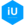Special Features
A collection that's special to our readers, contributors and us especially for special news.

# How to Find the GCF of Three or More Numbers?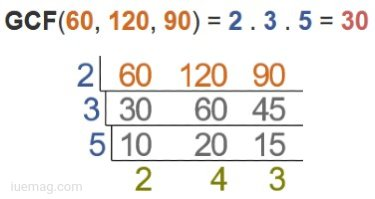GCF stands for greatest common factor. GCF is the study of finding the factors that exactly divide the number. It is widely used in mathematics. GCF is used in number theory and modular arithmetic. The working of GCF is very simple.

In this topic, we discuss the working of GCF and how to apply GCF on higher numbers of digits.

What is GCF?

GCF stands for greatest common factor. GCF of two are more numbers is the largest numbers among common factors of two or more numbers. GCF is based on common factors that are collected from given numbers and then identify the largest among them. GCF is also known as HCD (highest common divisor), HCF (highest common factor), or GCD (greatest common divisor).

How to calculate the GCF of Three or more numbers?

There are three ways to calculate GCF.

(i) List of multiples

(ii) Prime Factorization

(iii) Division method

Let’s find the GCF of three or more numbers by using these methods.

List of factors

In this method, we have to find the factors of two or more digits and then take the common factors among them and take the highest common factor.

Example 1

Find the GCF of 12, 16, and 24?

Solution

Step 1: Find the factors of these numbers.

Factors of 12 = 1, 2, 3, 4, 6, 12

Factors of 16 = 1, 2, 4, 8, 16

Factors of 24 = 1, 2, 3, 4, 6, 8, 12, 24

Step 2: Take the common factors among these numbers.

Common factors of 12, 16, and 24 = 1, 2, 4

Step 3: Choose the largest one among all common factors.

GCF of 12, 16, and 24 = 4

Example 2

Find the GCF of 15, 24, 36, and 45

Solution

Step 1: Find the factors of these numbers.

Factors of 15 = 1, 3, 5, 15

Factors of 24 = 1, 2, 3, 4, 6, 8, 12, 24

Factors of 36 = 1, 2, 3, 4, 6, 9, 12, 18, 36

Factors of 45 = 1, 3, 5, 9, 15, 45

Step 2: Take the common factors among these numbers.

Common factors of 15, 24, 36, and 45 = 1, 3

Step 3: Choose the largest one among all common factors.

GCF of 15, 24, 36, and 45 = 3

Example 3

Find the GCF of 15, 25, 35, 45, and 90?

Solution

Step 1: Find the factors of these numbers.

Factors of 15 = 1, 3, 5, 15

Factors of 25 = 1, 5, 25

Factors of 35 = 1, 5, 7, 35

Factors of 45 = 1, 3, 5, 9, 15, 45

Factors of 90 = 1, 2, 3, 5, 6, 9, 10, 15, 18, 30, 45, 90

Step 2: Take the common factors among these numbers.

Common factors of 15, 25, 35, 45, and 90 = 1, 5

Step 3: Choose the largest one among all common factors.

GCF of 15, 25, 35, 45, and 90 = 5

Prime Factorization

In this method, we have to find the prime factors of all the numbers and then take common factors and multiply all the common factors in order to find the GCF.

Example 1

Find the GCF of 24, 42, and 56 by using prime factorization?

Solution

Step 1: Find the prime factors of numbers.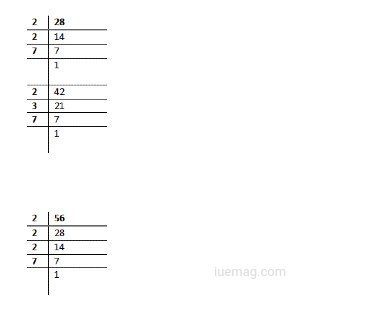Step 2: write all the prime factors.

Prime factorization of 24 = 2 x 2 x 7

Prime factorization of 42 = 2 x 3 x 7

Prime factorization of 56 = 2 x 2 x 2 x 7

Step 3: Take the common factors among these numbers.

Common prime factors = 2 x 7

Step 4: Multiply the common factors to get the GCF.

GCF = 2 x 7 = 14

Example 2

Find the GCF of 24, 48, 144, and 156 by using prime factorization?

Solution

Step 1: Find the prime factors of numbers.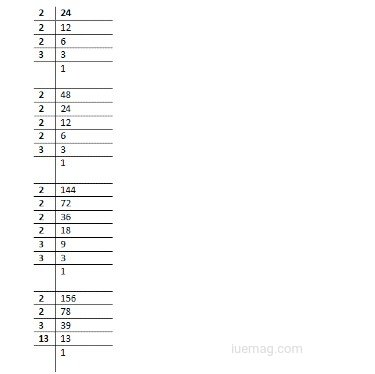Step 2: write all the prime factors.

Prime factorization of 24 = 2 x 2 x 2 x 3

Prime factorization of 48 = 2 x 2 x 2 x 2 x 3

Prime factorization of 144 = 2 x 2 x 2 x 2 x 3 x 3

Prime factorization of 156 = 2 x 2 x 3 x 13

Step 3: Take the common factors among these numbers.

Common prime factors = 2x2x3

Step 4: Multiply the common factors to get the GCF.

GCF = 2x2x3 = 12

Example 3

Find the GCF of 36, 48, 72, and 156 by using prime factorization?

Solution

Step 1: Find the prime factors of numbers.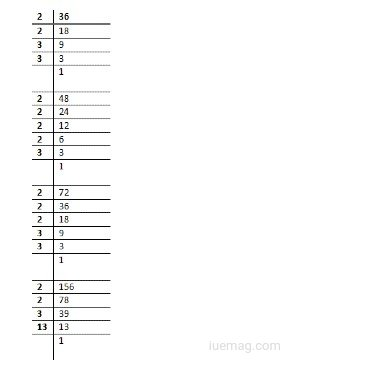Step 2: write all the prime factors.

Prime factorization of 34 = 2 x 2 x 3 x 3

Prime factorization of 48 = 2 x 2 x 2 x 2 x 3

Prime factorization of 72 = 2 x 2 x 2 x 3 x 3

Prime factorization of 156 = 2 x 2 x 3 x 13

Step 3: Take the common factors among these numbers.

Common prime factors = 2x2x3

Step 4: Multiply the common factors to get the GCF.

GCF = 2x2x3 = 12

You can use the GCF calculator in order to get the GCF calculations at one click.

### Division method

Example

Find the GCF of 126, 162, and 180 by using the division method.

Solution

Step 1:
First take two numbers. Take 126 as divisor and 162 as dividend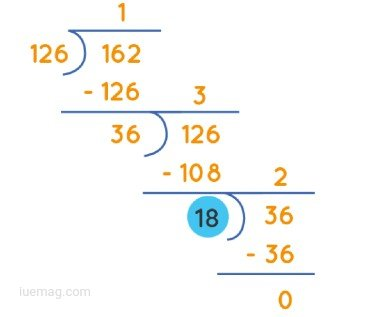Step 2:
identify the divisor which exactly divides the last number.

18

Step 3:
divide the third number by 18.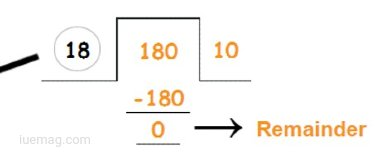Step 4:
The number which exactly divides all numbers is the GCF.

GCF = 18

The division method is not applicable for more than 3 values. The division method solves up to three numbers.

Summary
GCF is a number theory topic used to calculate the largest divisor of two or more numbers. GCF of two are more numbers is the largest numbers among common factors of two or more numbers. There are three methods to calculate GCF, a list of factors, prime factorization, and division method. The division method is only applicable for two or three numbers. The division method is not applicable for greater than three numbers.